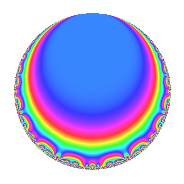# Properties

 Label 6048.2.a.bbLevel 6048 Weight 2 Character orbit 6048.a Self dual Yes Analytic conductor 48.294 Analytic rank 0 Dimension 2 CM No Inner twists 1

# Related objects

## Newspace parameters

 Level: $$N$$ = $$6048 = 2^{5} \cdot 3^{3} \cdot 7$$ Weight: $$k$$ = $$2$$ Character orbit: $$[\chi]$$ = 6048.a (trivial)

## Newform invariants

 Self dual: Yes Analytic conductor: $$48.2935231425$$ Analytic rank: $$0$$ Dimension: $$2$$ Coefficient field: $$\Q(\sqrt{2})$$ Coefficient ring: $$\Z[a_1, \ldots, a_{5}]$$ Coefficient ring index: $$2$$ Fricke sign: $$-1$$ Sato-Tate group: $\mathrm{SU}(2)$

## $q$-expansion

Coefficients of the $$q$$-expansion are expressed in terms of $$\beta = 2\sqrt{2}$$. We also show the integral $$q$$-expansion of the trace form.

 $$f(q)$$ $$=$$ $$q$$ $$+ ( -1 + \beta ) q^{5}$$ $$+ q^{7}$$ $$+O(q^{10})$$ $$q$$ $$+ ( -1 + \beta ) q^{5}$$ $$+ q^{7}$$ $$+ ( 2 + \beta ) q^{11}$$ $$+ \beta q^{13}$$ $$+ ( 3 - \beta ) q^{17}$$ $$+ ( 4 + \beta ) q^{19}$$ $$-4 q^{23}$$ $$+ ( 4 - 2 \beta ) q^{25}$$ $$+ \beta q^{29}$$ $$+ ( -4 - \beta ) q^{31}$$ $$+ ( -1 + \beta ) q^{35}$$ $$+ ( -3 + 2 \beta ) q^{37}$$ $$+ ( 1 + \beta ) q^{41}$$ $$+ ( 5 + \beta ) q^{43}$$ $$+ ( 3 + 2 \beta ) q^{47}$$ $$+ q^{49}$$ $$-2 q^{53}$$ $$+ ( 6 + \beta ) q^{55}$$ $$+ ( -5 + 2 \beta ) q^{59}$$ $$+ ( 6 - \beta ) q^{61}$$ $$+ ( 8 - \beta ) q^{65}$$ $$+ 4 q^{67}$$ $$+ ( -4 + 2 \beta ) q^{71}$$ $$-2 \beta q^{73}$$ $$+ ( 2 + \beta ) q^{77}$$ $$+ ( 1 - \beta ) q^{79}$$ $$+ ( -11 + 2 \beta ) q^{83}$$ $$+ ( -11 + 4 \beta ) q^{85}$$ $$-6 q^{89}$$ $$+ \beta q^{91}$$ $$+ ( 4 + 3 \beta ) q^{95}$$ $$+ ( -4 - 5 \beta ) q^{97}$$ $$+O(q^{100})$$ $$\operatorname{Tr}(f)(q)$$ $$=$$ $$2q$$ $$\mathstrut -\mathstrut 2q^{5}$$ $$\mathstrut +\mathstrut 2q^{7}$$ $$\mathstrut +\mathstrut O(q^{10})$$ $$2q$$ $$\mathstrut -\mathstrut 2q^{5}$$ $$\mathstrut +\mathstrut 2q^{7}$$ $$\mathstrut +\mathstrut 4q^{11}$$ $$\mathstrut +\mathstrut 6q^{17}$$ $$\mathstrut +\mathstrut 8q^{19}$$ $$\mathstrut -\mathstrut 8q^{23}$$ $$\mathstrut +\mathstrut 8q^{25}$$ $$\mathstrut -\mathstrut 8q^{31}$$ $$\mathstrut -\mathstrut 2q^{35}$$ $$\mathstrut -\mathstrut 6q^{37}$$ $$\mathstrut +\mathstrut 2q^{41}$$ $$\mathstrut +\mathstrut 10q^{43}$$ $$\mathstrut +\mathstrut 6q^{47}$$ $$\mathstrut +\mathstrut 2q^{49}$$ $$\mathstrut -\mathstrut 4q^{53}$$ $$\mathstrut +\mathstrut 12q^{55}$$ $$\mathstrut -\mathstrut 10q^{59}$$ $$\mathstrut +\mathstrut 12q^{61}$$ $$\mathstrut +\mathstrut 16q^{65}$$ $$\mathstrut +\mathstrut 8q^{67}$$ $$\mathstrut -\mathstrut 8q^{71}$$ $$\mathstrut +\mathstrut 4q^{77}$$ $$\mathstrut +\mathstrut 2q^{79}$$ $$\mathstrut -\mathstrut 22q^{83}$$ $$\mathstrut -\mathstrut 22q^{85}$$ $$\mathstrut -\mathstrut 12q^{89}$$ $$\mathstrut +\mathstrut 8q^{95}$$ $$\mathstrut -\mathstrut 8q^{97}$$ $$\mathstrut +\mathstrut O(q^{100})$$

## Embeddings

For each embedding $$\iota_m$$ of the coefficient field, the values $$\iota_m(a_n)$$ are shown below.

For more information on an embedded modular form you can click on its label.

Label $$\iota_m(\nu)$$ $$a_{2}$$ $$a_{3}$$ $$a_{4}$$ $$a_{5}$$ $$a_{6}$$ $$a_{7}$$ $$a_{8}$$ $$a_{9}$$ $$a_{10}$$
1.1
 −1.41421 1.41421
0 0 0 −3.82843 0 1.00000 0 0 0
1.2 0 0 0 1.82843 0 1.00000 0 0 0
 $$n$$: e.g. 2-40 or 990-1000 Significant digits: Format: Complex embeddings Normalized embeddings Satake parameters Satake angles

## Inner twists

This newform does not admit any (nontrivial) inner twists.

## Atkin-Lehner signs

$$p$$ Sign
$$2$$ $$-1$$
$$3$$ $$-1$$
$$7$$ $$-1$$

## Hecke kernels

This newform can be constructed as the intersection of the kernels of the following linear operators acting on $$S_{2}^{\mathrm{new}}(\Gamma_0(6048))$$:

 $$T_{5}^{2}$$ $$\mathstrut +\mathstrut 2 T_{5}$$ $$\mathstrut -\mathstrut 7$$ $$T_{11}^{2}$$ $$\mathstrut -\mathstrut 4 T_{11}$$ $$\mathstrut -\mathstrut 4$$ $$T_{13}^{2}$$ $$\mathstrut -\mathstrut 8$$ $$T_{17}^{2}$$ $$\mathstrut -\mathstrut 6 T_{17}$$ $$\mathstrut +\mathstrut 1$$